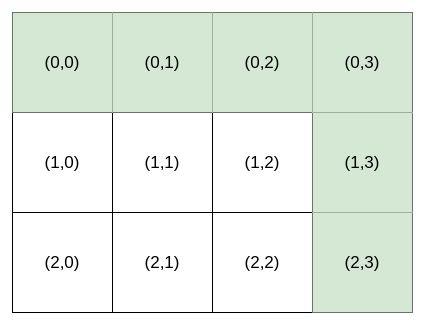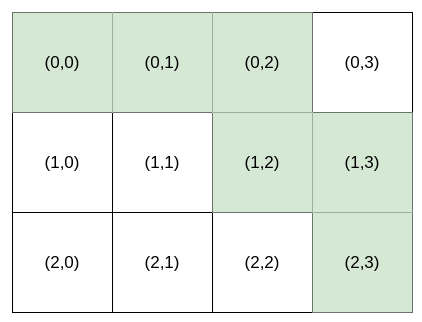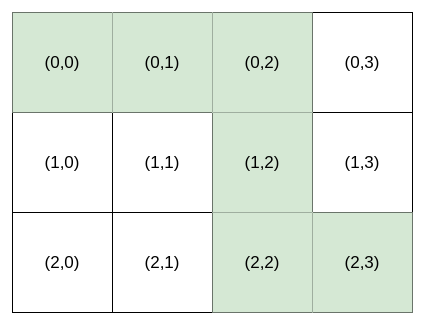# GeetCode Hub

Bob is standing at cell `(0, 0)`, and he wants to reach `destination`: `(row, column)`. He can only travel right and down. You are going to help Bob by providing instructions for him to reach `destination`.

The instructions are represented as a string, where each character is either:

• `'H'`, meaning move horizontally (go right), or
• `'V'`, meaning move vertically (go down).

Multiple instructions will lead Bob to `destination`. For example, if `destination` is `(2, 3)`, both `"HHHVV"` and `"HVHVH"` are valid instructions.

However, Bob is very picky. Bob has a lucky number `k`, and he wants the `kth` lexicographically smallest instructions that will lead him to `destination`. `k` is 1-indexed.

Given an integer array `destination` and an integer `k`, return the `kth` lexicographically smallest instructions that will take Bob to `destination`.

Example 1:```Input: destination = [2,3], k = 1
Output: "HHHVV"
Explanation: All the instructions that reach (2, 3) in lexicographic order are as follows:
["HHHVV", "HHVHV", "HHVVH", "HVHHV", "HVHVH", "HVVHH", "VHHHV", "VHHVH", "VHVHH", "VVHHH"].
```

Example 2:```Input: destination = [2,3], k = 2
Output: "HHVHV"
```

Example 3:```Input: destination = [2,3], k = 3
Output: "HHVVH"
```

Constraints:

• `destination.length == 2`
• `1 <= row, column <= 15`
• `1 <= k <= nCr(row + column, row)`, where `nCr(a, b)` denotes `a` choose `b`​​​​​.

class Solution { public String kthSmallestPath(int[] destination, int k) { } }